# DeepPose: Human Pose Estimation via Deep Neural Networks

DeepPose was proposed by researchers at Google for Pose Estimation in 2014 Computer Vision and Pattern Recognition conference. They work on formulating the pose Estimation problem as DNN-based regression problem towards body joints. They present a cascade of DNN-regressors which resulted in high precision pose estimates..

Architecture:

Pose Vector:

• To express the human body in the form of pose the authors of this paper encodes the location of all k body parts into joints called pose vector defined as following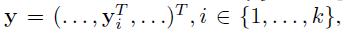• where yi  represents the x, y coordinates of  the  location of ith body joint.
• The image is represented in the form of (x, y) where x is image data and y is data of ground truth pose vector.
• Since, the coordinates described here are absolute image coordinates on full image size. If we resize image that may cause the problem. Therefore, we normalized the coordinates w.r.t a bounding box b which bounds the human body or some parts of it. These boxes are represented by b = (bc , bh , bw, ) where bc is the center of bounding box, bh is the height and bw is width of bounding.
• We normalized the location coordinates using following formula.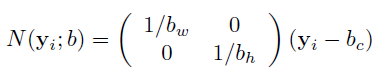• Finally we get the normalized Pose vector coordinates.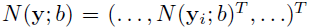CNN Architecture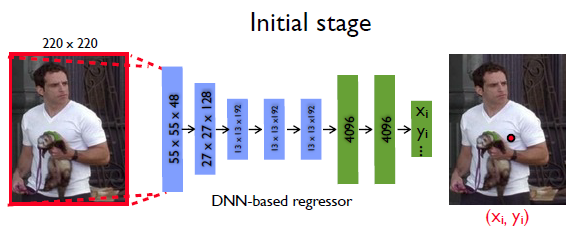• The authors of this paper uses AlexNet as their CNN architecture because it had shown great results on Image Localization task.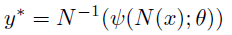• where theta represents the trainable parameters (weights and biases), shi represents the neural architecture applied to normalized pose vector N(x), The predicted output  y* can be obtained  by denormalization of output (N-1).
• This neural network architecture takes image of size 220×220 and apply an stride of 4.
• The CNN architecture contains 7 layers which can be listed as : C(55×55×96) — LRN — P — C(27×27×256) — LRN — P — C(13×13×384) — C(13×13×384) — C(13×13×256) — P — F(4096) — F(4096)
•  where C is convolution layer which uses ReLU as activation function to introduce non-linearity in the model, LRN is local response normalization, P is pooling layer, and F is fully connected layer.
• The last layer of architecture outputs 2k joint coordinates.
• Total number of parameters is 40 million.
• The architecture uses L2 loss function to minimize the distance between predicted coordinates and ground truth loss function.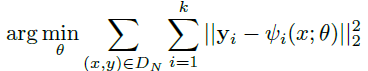• where k is the number of joints in the image

DNN regressor:

• It is not easy to increase the input size to have a finer pose estimation since this will increase the already large number of parameters. Thus, a cascade of pose regressors are proposed to refine the pose estimation.
• Now, we represent the first stage with following equation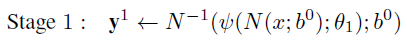• where b0 represents full image or bounding box obtained by a person detector.
• Now for the subsequent stages s>= 2: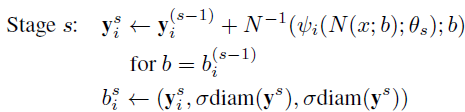• where diam(y) is the distance of opposing joints, such as left shoulder and right hips, and then scaled by ? to make it  ? diam(y).
• cascade of DNN regressor improved the accuracy as we can notice from the following images.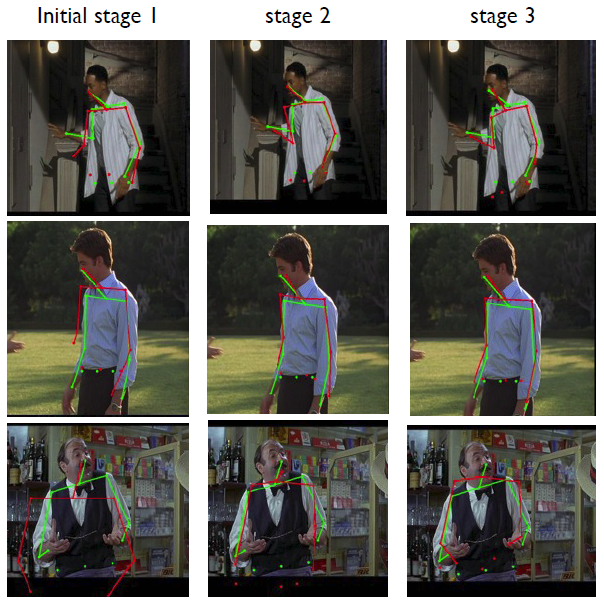Metrics:

• Percentage of Correct Parts (PCP) : It measures detection rate of limbs, where a limb is considered detected if the distance between the two predicted joint locations and the true limb joint locations is at most half of the limb length. However It has drawbacks like penalizing shorter and harder to detect limbs.
• Percent of Detected Joints (PDJ): To address the drawback caused by above method, another metric is proposed based on the detectiion of joints A joint is considered detected if the distance between the predicted and the true joint is within a certain fraction of the torso diameter. By varying this fraction, detection rates are obtained for varying degrees of localization precision.

Results:

• Framed Label In Cinema (FLIC) dataset :  This dataset contains 4000 train images with 1000 test images from Hollywood movies with different poses and clothing. For each labeled human, 10 upper body joints are labeled.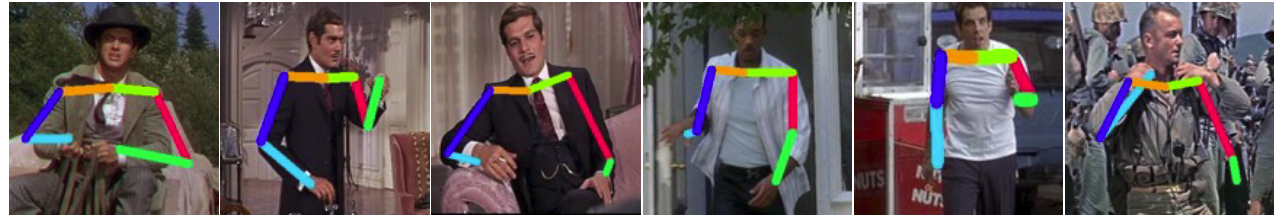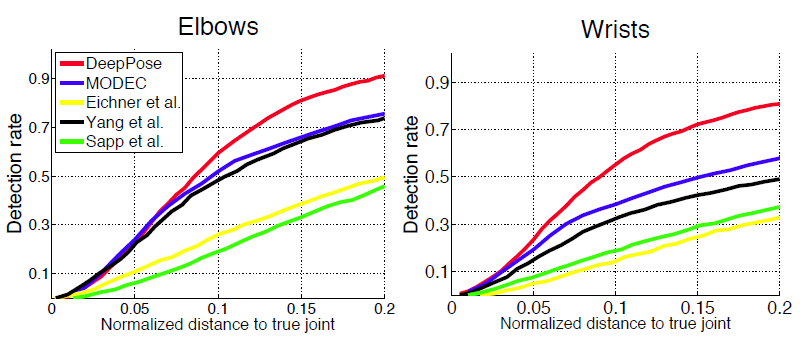• Leeds Sports Dataset (LSP): This dataset contains 11000 training and 1000 testing images from sports activities with challenging in terms of appearance and especially articulations. In this dataset, For each person the full body is labeled with total 14 joints.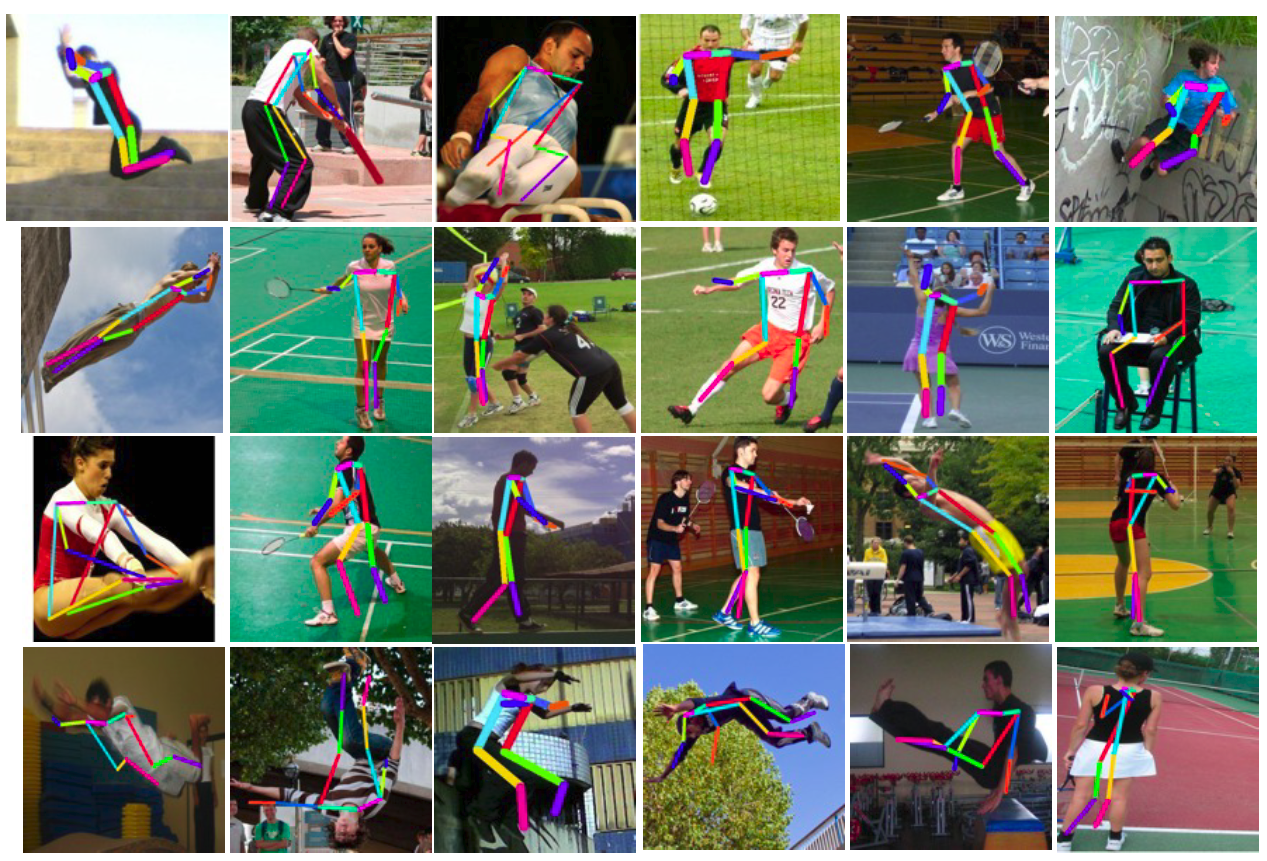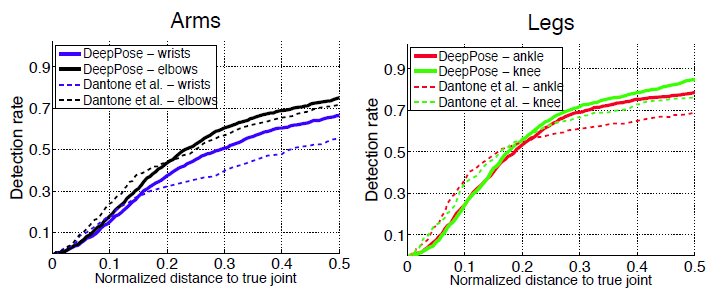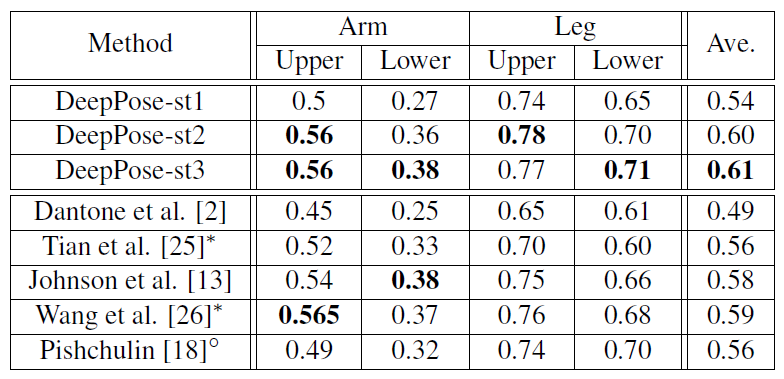• To address the generalization of the model trained on FLIC and LSP dataset, the performance is also evaluated on Buffy dataset and Image Parse dataset.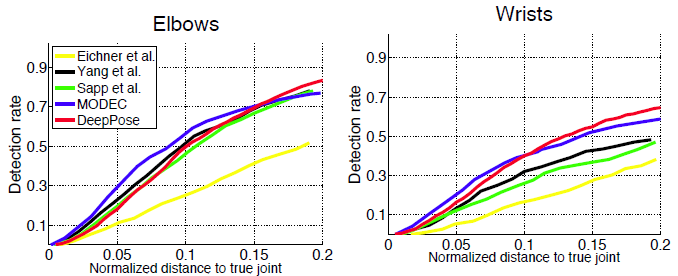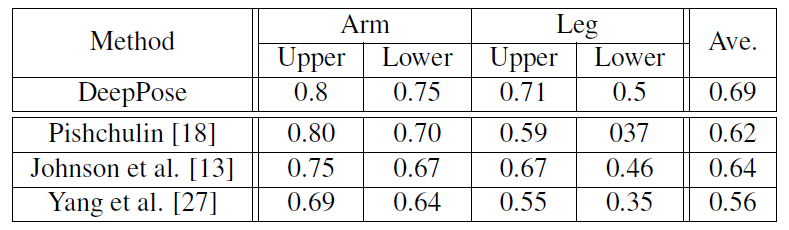References:

My Personal Notes arrow_drop_upCheck out this Author's contributed articles.

If you like GeeksforGeeks and would like to contribute, you can also write an article using contribute.geeksforgeeks.org or mail your article to contribute@geeksforgeeks.org. See your article appearing on the GeeksforGeeks main page and help other Geeks.

Please Improve this article if you find anything incorrect by clicking on the "Improve Article" button below.

Article Tags :
Practice Tags :

Be the First to upvote.

Please write to us at contribute@geeksforgeeks.org to report any issue with the above content.